In the last article we looked at how we could read configuration with a Kleisli Arrow similar to using a Reader Monad.

We’ve been using Arrows for the last couple of articles but haven’t defined what an Arrow is exactly.

An Arrow is a computation that runs within a context which takes in an input and returns an output. A more detailed explanation from Typeclassopedia states:

The Arrow class represents another abstraction of computation, in a similar vein to Monad and Applicative. However, unlike Monad and Applicative, whose types only reflect their output, the type of an Arrow computation reflects both its input and output. Arrows generalize functions: if arr is an instance of Arrow, a value of type b `arr` c can be thought of as a computation which takes values of type b as input, and produces values of type c as output. In the (->) instance of Arrow this is just a pure function; in general, however, an arrow may represent some sort of “effectful” computation

In Cats the Arrow typeclass is defined with the type constructor F which has two type holes:

``Arrow[F[_, _]] //simplified``

These two type holes correspond to the input and output types of the Arrow respectively. F can be any type constructor that takes two types and performs a mapping between them. A scala.Function1 is an example of F, as is the Kleisli Arrow we saw in previous articles. It might be helpful to think of Arrows as simple functions from one type to another for the moment.

Lets now go through some of the functions defined on Arrow and how they are used. For the remainder of the article lets assume that the type constructor supplied to Arrow is a scala.Function1:

``trait Function1[-T1, +R] extends AnyRef``

and the resulting Arrow is:

``val fa = Arrow[Function1]``

### lift/arr

This is a simple function to construct an Arrow given its input and output types. This is defined in Cats as:

``def lift[A, B](f: A => B): F[A, B]``

For example to lift a function that goes from a String to an Int into F we’d do:

``````val findLength: String => Int = _.length
fa.lift(f) //Function1[String, Int]``````

Since findLength is already a scala.Function1 it is a little pointless to lift it into a scala.Function1 but hopefully its usage is clear.

In Scalaz this function is defined as arr:

``def arr[A, B](f: A => B): A =>: B``

where =>: is a typeconstructor similar to F.

### id

The id function is defined as:

``def id[A]: F[A, A]``

The type signature of the above tells us that F returns the input type A as its output, essentially giving us the identity function.

``````val intF1 = fa.id[Int] //Function1[Int, Int]
intF1(10) //returns 10``````

### first

The first function is defined as:

``def first[A, B, C](fa: F[A, B]): F[(A, C), (B, C)]``

The first function takes Arrow fa from A => B and returns another Arrow (A, C) => (B, C). It applies the function in fa to the first parameter of the tuple, which is an A and converts it to a B. The second parameter of the tuple it leaves untouched and returns a (B, C).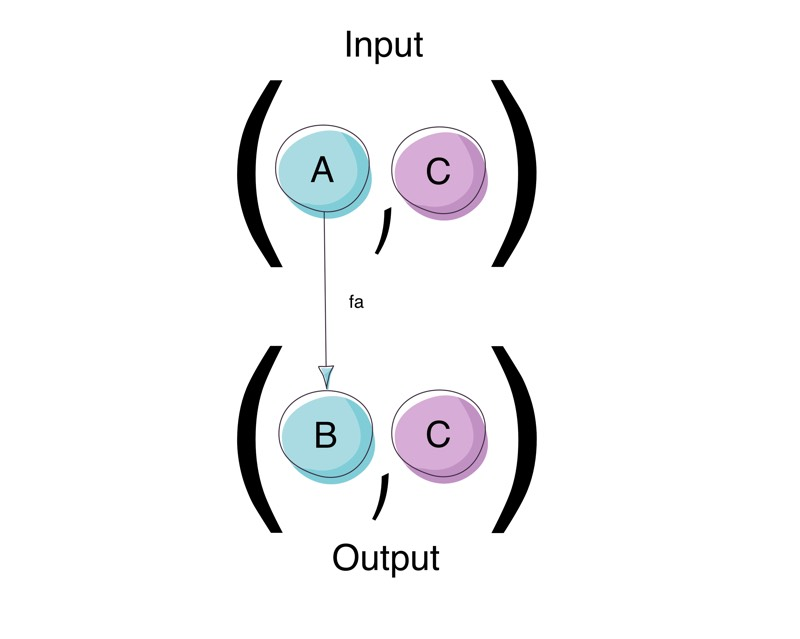First

For the remaining examples we have the following definitions at our disposal:

``````final case class Name(first: String, last: String)
final case class Age(age: Int)
final case class Person(name: Name, age: Age)

val name = Name("Nagate", "Tanikaze")
val age = Age(22)

def upperFirstName: String => String = _.toUpperCase
def doubleNumber: Int => Int = _ * 2

def upperName: Name => Name = n => Name(upperFirstName(n.first), n.last)
def doubleAge: Age => Age = a => Age(doubleNumber(a.age))``````

For example if we wanted to apply a function to the Name element of a Name and Age pair and but wanted to leave the Age element untouched we could do:

``````val onlyNameF: ((Name, Age)) => (Name, Age) = fa.first[Name, Name, Age](upperName)
val toPersonF: ((Name, Age)) => Person = onlyNameF andThen (Person.apply _).tupled
toPersonF(name, age) //returns Person(Name(NAGATE,Tanikaze),Age(22))``````

Notice how the Age value of the input is unchanged.

### second

The second function is very similar to first only with its parameters switched. It is defined as:

``def second[A, B, C](fa: F[A, B]): F[(C, A), (C, B)]``

The second function takes Arrow fa from A => B and returns another Arrow with takes in a tuple of (C, A) => (C, B). It applies the function in fa to the second parameter of the tuple A and converts it to a B. The first parameter of the tuple it leaves untouched and returns a (C, B).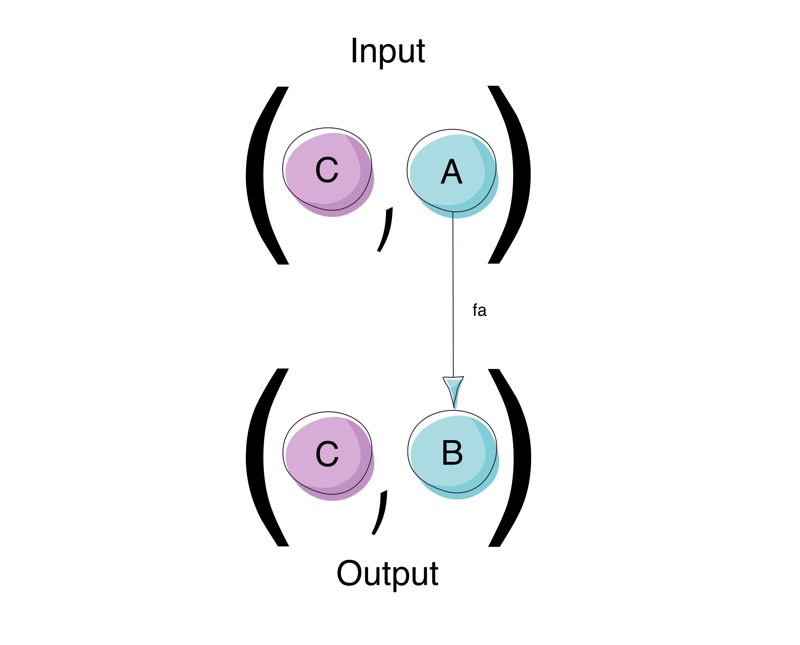Second

For example if we wanted to apply a function to the Age element of a Name and Age pair and but wanted to leave the Name element untouched we could do:

``````val onlyAgeF: ((Name, Age)) => (Name, Age) = fa.second[Age, Age, Name](doubleAge)
val toPersonF: ((Name, Age)) => Person = onlyAgeF andThen (Person.apply _).tupled
toPersonF(name, age) //returns Person(Name(Nagate,Tanikaze),Age(44))``````

Notice how the Name value of the input is unchanged.

### split/product/***

The split function is an application of first and second. It is defined as:

``def split[A, B, C, D](f: F[A, B], g: F[C, D]): F[(A, C), (B, D)]``

The split function takes Arrow f from A => B and an Arrow g from C => D and returns another Arrow with takes in a tuple of (A, C) => (B, D). It applies the function in f to the first parameter of the tuple A and converts it to a B. It also applies the function in g to the second parameter of the tuple C and converts it to a D returning a final result of (B, D). Split has the symbolic representation of *** and is sometimes referred to as the product function because it applies multiple functions to multiple inputs.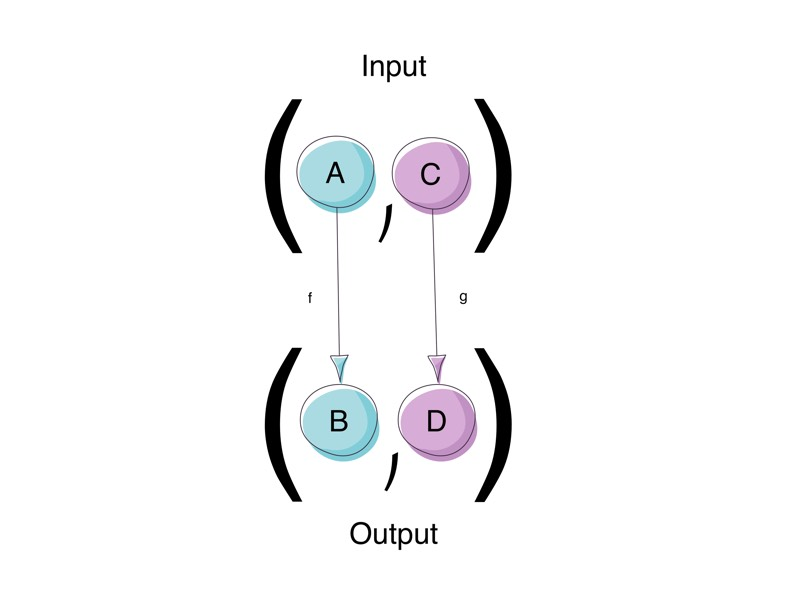Split

For example if we wanted to apply a function to the Name and Age element of a Name and Age pair at once we could do:

``````val bothNameAndAgeF: ((Name, Age)) => (Name, Age) = fa.split[Name, Name, Age, Age](upperName, doubleAge)
val toPersonF: ((Name, Age)) => Person = bothNameAndAgeF andThen (Person.apply _).tupled
toPersonF(name, age)//Person(Name(NAGATE,Tanikaze),Age(44))``````

### combine/fanout/&&&

combine is defined as:

``def combine[A, B, C](fab: F[A, B], fac: => F[A, C]): F[A, (B, C)]``

Although Cats does not define combine, scalaz does. For the purpose of this post I’ve created an implementation of combine in the example source.

The combine function takes Arrow fab from A => B and an Arrow fac from A => C and returns another Arrow which takes in an input of A, and returns a tuple of (B, C). It’s important to note that the same input A is supplied to both arrows fab and fac.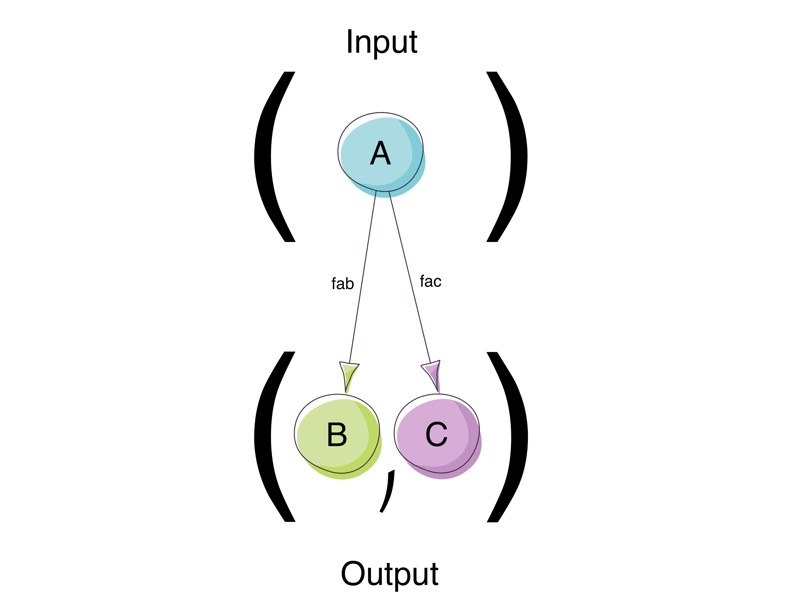Combine

For example given a Person if we want to break it into primitive representations of its Name and Age fields we could do:

``````val person = Person(name, age)
val combineName: Person => String = {
case Person(Name(first, last), _) => s"\$first \$last"
}
val combineAge: Person => Int = _.age.age
val combineF: Person => (String, Int) = ArrowFuncs.combine(combineName, combineAge)
combineF(person) // ("Nagate Tanikaze",22): (String, Int)``````

combine has a symbolic representation of &&& and is sometimes referred to as the fanout function.

### liftA2

liftA2 is defined as:

``def liftA2[A, B, C, D](fab: F[A, B], fac: F[A, C])(f: B => C => D): F[A, D] //simplified``

I could not find a definition of liftA2 in either Cats nor Scalaz. I’ve referenced it here directly from the Generalising monads to arrows paper by John Hughes in Haskell:

``liftA2 :: Arrow a => (b -> c -> d) -> a e b -> a e c -> a e d``

A sample of implementation of this can be found in the example source.

The liftA2 function is very similar to the combine function with the addition of running a function on the result of combine.

The liftA2 function takes an Arrow fab from A => B, an Arrow fac from A => C and a function f from B => C => D and returns another Arrow with takes in an input of A, and returns a D.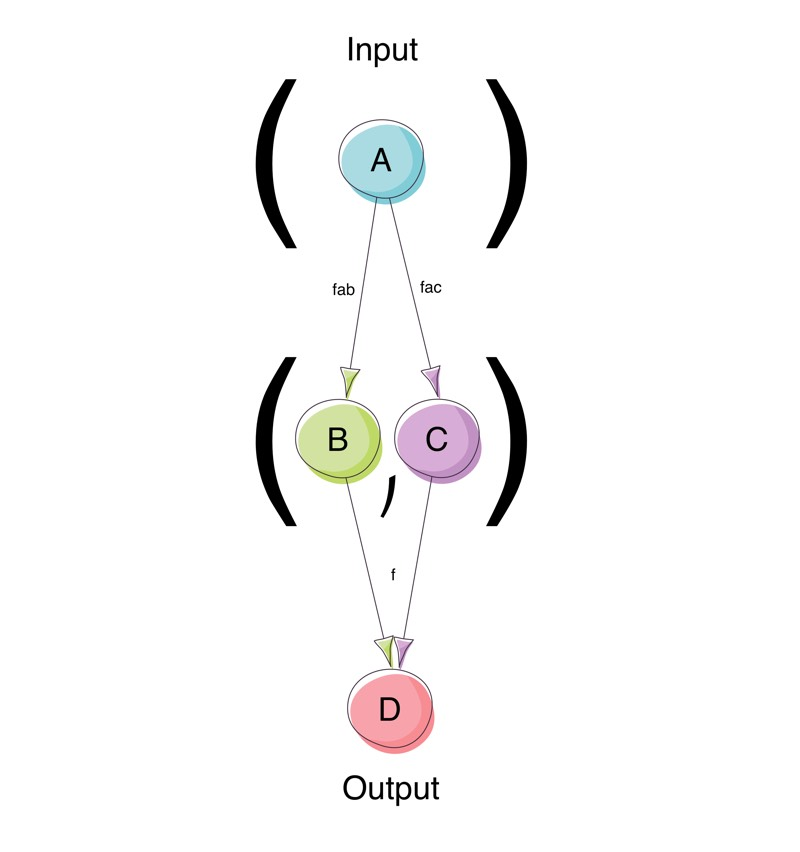liftA2

For example given a Person if we want to break it into primitive representations of its Name and Age fields and then apply a function on the separated bits we could do:

``````val person = Person(name, age)
val combineName: Person => String = {
case Person(Name(first, last), _) => s"\$first \$last"
}
val combineAge: Person => Int = _.age.age
def makePersonString: String => Int => String = name => age => s"person[name='\$name', age=\$age]"
val lifta2: Person => String = ArrowFuncs.liftA2(combineName, combineAge)(makePersonString)
lifta2(person) //"person[name='Nagate Tanikaze', age=22]"``````

### compose/<<< and andThen/>>>

compose is defined as:

``def compose[A, B, C](f: F[B, C], g: F[A, B]): F[A, C]``

and has the symbolic representation of <<<.

andThen is defined as:

``def andThen[A, B, C](f: F[A, B], g: F[B, C]): F[A, C]``

and has the symbolic representation of >>>.

compose and andThen are basically the same function with the first and second arguments swapped.

These functions combine arrows passing on the output of one arrow as the input to the next one, similar to regular function composition.

For example given a Name and Age, if we wanted to convert them to a Person and then covert the Person to a String we could do:

``````def personA: Tuple2[Name, Age] => Person = na => Person(na._1, na._2)
val makePersonStringA: Person  => String = p =>  s"person[name='\${p.name.first}' \${p.name.last}, age=\${p.age} yrs]"

val composeF: Tuple2[Name, Age] => String = personA >>> makePersonStringA
val andThenF: Tuple2[Name, Age] => String =  makePersonStringA <<< personA

composeF(name, age) //person[name='Nagate' Tanikaze, age=Age(22) yrs]
andThenF(name, age) //person[name='Nagate' Tanikaze, age=Age(22) yrs]``````

### A Worked Example

We’ve learned a lot of functions which are somewhat cryptic until you start to use them. To make their usage a little clearer lets look at an example.

Assume we have the following functions at our disposal:

``````  final case class ItemId(id: Long)
final case class ItemDescReq(itemId: Long, userId: String)
final case class ItemDetail(itemId: Long, value: Double, desc: String)
final case class User(name: String, id: String)
final case class ValuableItemsResponse(expensive: List[ItemDetail], veryExpensive: List[ItemDetail])
final case class Price(price: Double)

type UserData = Map[String, List[ItemId]]
type ItemData = Map[Long, ItemDetail]
type Results  = List[Either[String, ItemDetail]]

val userData = Map[String, List[ItemId]](
"1000" -> List(ItemId(1001), ItemId(1002), ItemId(1003), ItemId(1007), ItemId(1004)),
"2000" -> List(ItemId(2001), ItemId(2002))
)

val itemData = Map[Long, ItemDetail](
1001L -> ItemDetail(1001, 2000.00,  "Couch"),
1002L -> ItemDetail(1002, 100.00,   "Apple TV"),
1003L -> ItemDetail(1003, 75000.00, "Luxury Car"),
1004L -> ItemDetail(1004, 3000,     "Laptop"),
2001L -> ItemDetail(2001, 1500.00,  "Coffee Machine"),
2002L -> ItemDetail(2002, 500.00,   "DLSR")
)

val getSavedItems: User => UserData => List[ItemId] = user => data => data.getOrElse(user.id, Nil)

val idToDesc: User => ItemId => ItemDescReq = user => itemId => ItemDescReq(itemId.id, user.id)

val getDetails: ItemDescReq => ItemData => Either[String, ItemDetail] = itemDescReq => data =>
data.get(itemDescReq.itemId).toRight(s"could not find item with id: \${itemDescReq.itemId}")

val isExpensive: Range => ItemDetail => Boolean = range => item => range.contains(item.value)

val valuableItemsResponse : Tuple2[Range, Range] => List[ItemDetail] => ValuableItemsResponse = prices => items =>
ValuableItemsResponse(items.filter(isExpensive(prices._1)), items.filter(isExpensive(prices._2)))

val valuableItemsResponseString: ValuableItemsResponse => String = items => {
s"expensive:\${itemDetailString(items.expensive)},veryExpensive:\${itemDetailString(items.veryExpensive)}"
}

val itemDetailString: List[ItemDetail] => String = _.map(id => s"\${id.desc}=\$\$\${id.value}").mkString(",")

val errorString: List[Either[String, ItemDetail]] => String = itemsE =>
itemsE.collect { case Left(error) => error } mkString("\n")``````

We now want to use the above functions to do the following:

1. Get the saved items for a User.
2. Convert each item to a item request.
3. Look up the details of each item requested. (this may fail)
4. Filter the successful requests against two price ranges, one for expensive and the other for very expensive.
5. The filtered items should then be put into a ValuableItemsResponse object.
6. At the end we need to print out a description of the valuable items found and any errors that were generated.

We can then glue these functions together to give us the output we desire:

``````  // User => (UserData => List[ItemId], (ItemId => ItemDescReq))
val f1 = ArrowFuncs.combine(getSavedItems, idToDesc)

// User => List[ItemDescReq]
val f2 = f1 >>> { case (fi, fd) =>  fi(userData) map fd }

//User => (Results, Results)
val f3 = f2 >>> (_ map getDetails) >>> (_ map (_(itemData))) >>> (_.partition(_.isLeft))

//(Results, Results) => (Results, List[ItemDetail])
val f4 = fa.second[Results, List[ItemDetail], Results](_ collect { case Right(value) => value })

//User => (Results, List[ItemDetail])
val f5 = f3 >>> f4

//(Results, List[ItemDetail]) => (Results, Tuple2[Range, Range] => ValuableItemsResponse)
val f6 =
fa.second[List[ItemDetail],
Tuple2[Range, Range] => ValuableItemsResponse,
Results](
items => prices => valuableItemsResponse(prices)(items)
)

//User => (Results, Tuple2[Range, Range] => ValuableItemsResponse)
val f7 = f5 >>>  f6

//(Results, Tuple2[Range, Range] => ValuableItemsResponse) => (Results, ValuableItemsResponse)
val f8 =
fa.second[
Tuple2[Range, Range] => ValuableItemsResponse,
ValuableItemsResponse,
Results](_(Range(500, 3000), Range(10000, 100000)))

//User => (Results, ValuableItemsResponse)
val f9 = f7 >>> f8

//(Results, ValuableItemsResponse) => (String, String)
val f10 = fa.split[Results, String, ValuableItemsResponse, String](
errorString, valuableItemsResponseString
)

//User => (String, String)
val f11 = f9 >>> f10

val (errors, values) = f11(User("Guybrush threepwood", "1000"))``````

which outputs:

``expensive:Couch=\$2000.0,veryExpensive:Luxury Car=\$75000.0, errors: could not find item with id: 1007``

or more succinctly:

``````val pipeline =
ArrowFuncs.combine(getSavedItems, idToDesc) >>>
{ case (fi, fd) =>  fi(userData) map fd } >>>
(_ map getDetails) >>>
(_ map (_(itemData))) >>>
(_.partition(_.isLeft)) >>>
fa.second[Results, List[ItemDetail], Results](_ collect { case Right(value) => value }) >>>
fa.second[List[ItemDetail], Tuple2[Range, Range] => ValuableItemsResponse, Results](
items => prices => valuableItemsResponse(prices)(items)
) >>>
fa.second[Tuple2[Range, Range] => ValuableItemsResponse, ValuableItemsResponse, Results](
_(Range(500, 3000), Range(10000, 100000))
) >>>
fa.split[Results, String, ValuableItemsResponse, String](
errorString, valuableItemsResponseString
)

val (errors, values) = pipeline(User("Guybrush threepwood", "1000"))``````

Hopefully this has given you a gentle introduction into the world of Arrows.